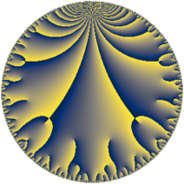# Properties

 Label 98.10.aLevel $98$ Weight $10$ Character orbit 98.a Rep. character $\chi_{98}(1,\cdot)$ Character field $\Q$ Dimension $31$ Newform subspaces $12$ Sturm bound $140$ Trace bound $3$

# Related objects

## Defining parameters

 Level: $$N$$ $$=$$ $$98 = 2 \cdot 7^{2}$$ Weight: $$k$$ $$=$$ $$10$$ Character orbit: $$[\chi]$$ $$=$$ 98.a (trivial) Character field: $$\Q$$ Newform subspaces: $$12$$ Sturm bound: $$140$$ Trace bound: $$3$$ Distinguishing $$T_p$$: $$3$$

## Dimensions

The following table gives the dimensions of various subspaces of $$M_{10}(\Gamma_0(98))$$.

Total New Old
Modular forms 134 31 103
Cusp forms 118 31 87
Eisenstein series 16 0 16

The following table gives the dimensions of the cuspidal new subspaces with specified eigenvalues for the Atkin-Lehner operators and the Fricke involution.

$$2$$$$7$$FrickeDim.
$$+$$$$+$$$$+$$$$7$$
$$+$$$$-$$$$-$$$$8$$
$$-$$$$+$$$$-$$$$9$$
$$-$$$$-$$$$+$$$$7$$
Plus space$$+$$$$14$$
Minus space$$-$$$$17$$

## Trace form

 $$31 q + 16 q^{2} + 6 q^{3} + 7936 q^{4} + 756 q^{5} - 544 q^{6} + 4096 q^{8} + 164295 q^{9} + O(q^{10})$$ $$31 q + 16 q^{2} + 6 q^{3} + 7936 q^{4} + 756 q^{5} - 544 q^{6} + 4096 q^{8} + 164295 q^{9} - 57344 q^{10} + 47360 q^{11} + 1536 q^{12} - 149328 q^{13} - 574204 q^{15} + 2031616 q^{16} + 1133226 q^{17} + 817616 q^{18} - 364822 q^{19} + 193536 q^{20} + 310208 q^{22} + 381988 q^{23} - 139264 q^{24} + 19653841 q^{25} - 2801728 q^{26} + 1068372 q^{27} + 316342 q^{29} + 4605312 q^{30} - 2041188 q^{31} + 1048576 q^{32} - 41272000 q^{33} - 9521056 q^{34} + 42059520 q^{36} + 61320506 q^{37} + 18670112 q^{38} + 33141280 q^{39} - 14680064 q^{40} - 36076902 q^{41} - 161828564 q^{43} + 12124160 q^{44} + 112432292 q^{45} + 39999872 q^{46} - 24343308 q^{47} + 393216 q^{48} + 130681520 q^{50} - 3882188 q^{51} - 38227968 q^{52} - 89805274 q^{53} - 35188672 q^{54} + 12306224 q^{55} + 283722084 q^{57} - 199875936 q^{58} - 3712782 q^{59} - 146996224 q^{60} - 43059924 q^{61} - 111019072 q^{62} + 520093696 q^{64} + 124521768 q^{65} + 114458624 q^{66} + 176737592 q^{67} + 290105856 q^{68} + 286188224 q^{69} + 335540328 q^{71} + 209309696 q^{72} - 113029370 q^{73} + 97442592 q^{74} + 1419702850 q^{75} - 93394432 q^{76} + 315704192 q^{78} - 375697700 q^{79} + 49545216 q^{80} + 997890439 q^{81} + 732392544 q^{82} + 591878946 q^{83} + 1429760996 q^{85} + 879180224 q^{86} + 1233966460 q^{87} + 79413248 q^{88} - 124823298 q^{89} - 2088905728 q^{90} + 97788928 q^{92} - 1867544788 q^{93} - 1081162944 q^{94} - 332382196 q^{95} - 35651584 q^{96} + 792186490 q^{97} + 1215212156 q^{99} + O(q^{100})$$

## Decomposition of $$S_{10}^{\mathrm{new}}(\Gamma_0(98))$$ into newform subspaces

Label Dim $A$ Field CM Traces A-L signs $q$-expansion
$a_{2}$ $a_{3}$ $a_{5}$ $a_{7}$ 2 7
98.10.a.a $1$ $50.474$ $$\Q$$ None $$-16$$ $$6$$ $$-560$$ $$0$$ $+$ $-$ $$q-2^{4}q^{2}+6q^{3}+2^{8}q^{4}-560q^{5}+\cdots$$
98.10.a.b $1$ $50.474$ $$\Q$$ None $$16$$ $$-170$$ $$-544$$ $$0$$ $-$ $-$ $$q+2^{4}q^{2}-170q^{3}+2^{8}q^{4}-544q^{5}+\cdots$$
98.10.a.c $1$ $50.474$ $$\Q$$ None $$16$$ $$156$$ $$-870$$ $$0$$ $-$ $-$ $$q+2^{4}q^{2}+156q^{3}+2^{8}q^{4}-870q^{5}+\cdots$$
98.10.a.d $2$ $50.474$ $$\Q(\sqrt{43})$$ None $$-32$$ $$0$$ $$0$$ $$0$$ $+$ $-$ $$q-2^{4}q^{2}+\beta q^{3}+2^{8}q^{4}+35\beta q^{5}+\cdots$$
98.10.a.e $2$ $50.474$ $$\Q(\sqrt{2305})$$ None $$-32$$ $$14$$ $$2730$$ $$0$$ $+$ $-$ $$q-2^{4}q^{2}+(7+5\beta )q^{3}+2^{8}q^{4}+(1365+\cdots)q^{5}+\cdots$$
98.10.a.f $2$ $50.474$ $$\Q(\sqrt{106})$$ None $$32$$ $$0$$ $$0$$ $$0$$ $-$ $-$ $$q+2^{4}q^{2}+3\beta q^{3}+2^{8}q^{4}-31\beta q^{5}+\cdots$$
98.10.a.g $3$ $50.474$ $$\mathbb{Q}[x]/(x^{3} - \cdots)$$ None $$-48$$ $$-71$$ $$1085$$ $$0$$ $+$ $+$ $$q-2^{4}q^{2}+(-24-\beta _{1})q^{3}+2^{8}q^{4}+\cdots$$
98.10.a.h $3$ $50.474$ $$\mathbb{Q}[x]/(x^{3} - \cdots)$$ None $$-48$$ $$71$$ $$-1085$$ $$0$$ $+$ $-$ $$q-2^{4}q^{2}+(24+\beta _{1})q^{3}+2^{8}q^{4}+(-363+\cdots)q^{5}+\cdots$$
98.10.a.i $3$ $50.474$ $$\mathbb{Q}[x]/(x^{3} - \cdots)$$ None $$48$$ $$-233$$ $$-733$$ $$0$$ $-$ $-$ $$q+2^{4}q^{2}+(-78-\beta _{1})q^{3}+2^{8}q^{4}+\cdots$$
98.10.a.j $3$ $50.474$ $$\mathbb{Q}[x]/(x^{3} - \cdots)$$ None $$48$$ $$233$$ $$733$$ $$0$$ $-$ $+$ $$q+2^{4}q^{2}+(78+\beta _{1})q^{3}+2^{8}q^{4}+(246+\cdots)q^{5}+\cdots$$
98.10.a.k $4$ $50.474$ $$\Q(\sqrt{2}, \sqrt{4817})$$ None $$-64$$ $$0$$ $$0$$ $$0$$ $+$ $+$ $$q-2^{4}q^{2}+(5\beta _{1}+\beta _{3})q^{3}+2^{8}q^{4}+(104\beta _{1}+\cdots)q^{5}+\cdots$$
98.10.a.l $6$ $50.474$ $$\mathbb{Q}[x]/(x^{6} - \cdots)$$ None $$96$$ $$0$$ $$0$$ $$0$$ $-$ $+$ $$q+2^{4}q^{2}+(\beta _{2}+\beta _{3})q^{3}+2^{8}q^{4}+(-2\beta _{2}+\cdots)q^{5}+\cdots$$

## Decomposition of $$S_{10}^{\mathrm{old}}(\Gamma_0(98))$$ into lower level spaces

$$S_{10}^{\mathrm{old}}(\Gamma_0(98)) \cong$$ $$S_{10}^{\mathrm{new}}(\Gamma_0(2))$$$$^{\oplus 3}$$$$\oplus$$$$S_{10}^{\mathrm{new}}(\Gamma_0(7))$$$$^{\oplus 4}$$$$\oplus$$$$S_{10}^{\mathrm{new}}(\Gamma_0(14))$$$$^{\oplus 2}$$$$\oplus$$$$S_{10}^{\mathrm{new}}(\Gamma_0(49))$$$$^{\oplus 2}$$